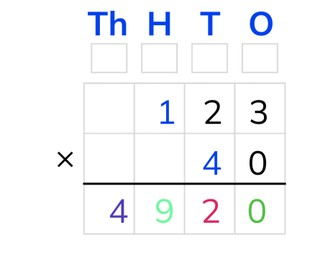Standard algorithm multiplication with tens and numbers to 1,000

# Standard algorithm multiplication with tens and numbers to 1,000

Students learn standard algorithm multiplication with tens and numbers to 1,000.

No account needed.8,000 schools use Gynzy92,000 teachers use Gynzy1,600,000 students use Gynzy

## General

The students learn standard algorithm multiplication with a tens number and a number to 1,000.

## Standards

CCSS.Math.Content.5.NBT.B.5

## Learning objective

Students will be able to multiply using the standard algorithm with a tens number and a number to 1,000.

## Introduction

The students solve problems for which they must multiply using the standard algorithm.

## Instruction

Explain that with standard algorithm multiplication you start by putting the numbers one under the other. You put the largest number on top. The T, H, T, O above the table refer to the thousands, hundreds, tens, and ones. Show that above each column there is a box for regrouping. In these regrouping boxes your can write down numbers that are regrouped to add them up later with the other outcomes. Explain that you start with the lower number on the right in the ones column. Emphasize that with standard algorithm multiplication with a tens number you can already write down a zero, because all multiplication problems with 0 have an outcome of 0. Now multiply the lower number from the tens (T) column with the higher number from the ones (O) column (4 × 3 = 12). Of this 12 you write the 2 down under the line in the tens (T) column. You write the 1 (10) in the regrouping box of the hundreds. Next you multiply the lower number from the tens (T) column with the higher number from the tens (T) column (4 × 2 =8). Emphasize that you shouldn't forget to add on the 1 from the regrouping box (8 + 1 = 9). You write the 9 under the line with the hundreds numbers. Now you multiply the lower number from the tens (T) column with the higher number from the hundreds (H) column (4 × 1 = 4). The outcome is 4,920. Practice two problems together with the students which must be solved using the standard algorithm. Students may use scrap paper. Explain that with problems with larger numbers you will also need columns for the hundred- and ten-thousands. Using the steps, show that the standard algorithm is applied in the same way. Emphasize that depending on the numbers you are multiplying you may have to use more of the regrouping boxes. The students solve the next two problems on their own.

Check whether the students can multiply using the standard algorithm multiplication with a tens number and a number to 1,000 by asking the following question:
- What steps do you take to solve the problem 209 × 20 using the standard algorithm?

## Quiz

The students test their understanding of standard algorithm multiplication with tens and numbers to 1,000 through ten exercises. For some of the problems the numbers are already filled in on the chart, and for others the students must fill in the chart themselves.

## Closing

Discuss once again the importance of being able to multiply using the standard algorithm with a tens number and a number to 1,000. As a closing activity you can have the students solve the problems in pairs, for which they must give the answer in apples. To check the apples can be dragged to the chart. As an extra assignment the students can make a problem in apples on their own and have their partner solve it.

## Teaching tips

Have students that have difficulty with standard algorithm multiplication with a tens number and a number to 1,000 first practice with standard algorithm multiplication with numbers to 100. Emphasize that the students can use the regrouping boxes.

## Instruction materials

Scrap paper

### The online teaching platform for interactive whiteboards and displays in schools

• Save time building lessons

• Manage the classroom more efficiently

• Increase student engagement